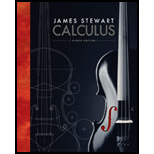# Suppose u and v are vector functions that possess limits as t → a and let c be a constant. Prove the following properties of limits. (a) lim t → a [ u ( t ) + v ( t ) ] = lim t → a u ( t ) + lim t → a v ( t ) (b) lim t → a c u ( t ) = c lim t → a u ( t ) (c) lim t → a [ u ( t ) ⋅ v ( t ) ] = lim t → a u ( t ) ⋅ lim t → a v ( t ) (d) lim t → a [ u ( t ) × v ( t ) ] = lim t → a u ( t ) × lim t → a v ( t )BuyFind

### Calculus (MindTap Course List)

8th Edition
James Stewart
Publisher: Cengage Learning
ISBN: 9781285740621BuyFind

### Calculus (MindTap Course List)

8th Edition
James Stewart
Publisher: Cengage Learning
ISBN: 9781285740621

#### Solutions

Chapter 13.1, Problem 53E
Textbook Problem

## Expert Solution

### Want to see the full answer?

Check out a sample textbook solution.

### Want to see this answer and more?

Experts are waiting 24/7 to provide step-by-step solutions in as fast as 30 minutes!*

*Response times vary by subject and question complexity. Median response time is 34 minutes and may be longer for new subjects.© 2021 bartleby. All Rights Reserved.